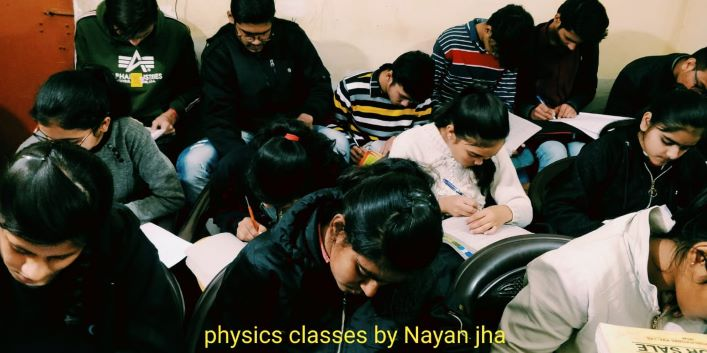# Sample paper  for class -12th (physics)

This is the first sample paper designed on the basis of latest CBSE pattern , by physics classes by nayan jha. student may solve the entire question paper for  the practice well before the examination. I also want to inform that such kind of question paper will be provided every week ( on sunday) student can download all question paper and test himself after solving all question papers.

Sample paper -1 .Sec-A   mark-1

1. Two charges 3×10-5 and 5×104C are placed at a distance 10cm from each other. Find the value of electrostatic force acting between them.                                (a)5×1011N(b)40×1011N(c)180×109N(d)5×1010N
2.  When charge is supplied to a conductor, its potential depends upon                                                        (a)The amount of charge  (b)Geometry and size of conductor        (c)Both (a) & (b)         (d)Only on (a)
3. Kirchhoff’s II law for the electric network is based on:                                                                                 (a)Law of conversation of charge  (b)Low of conversation of energy    (c)Low of conversation of angular momentum    (d)Low of conversation of mass

1. In a whetstone’s bridge, all the four arms have equal resistance R. if resistance of the galvanometer arm is also R, then equivalent resistance of combination is              (a)R(b)2R(c)R/2(d)R/4

1. In a certain reason of space, electric filled E vector and magnetic filled b vector are perpendicular to each other. An electron inters perpendicularly to both the fields and move undeflected. The velocity of electron is                                                                                                                                           (a)E/B    (b)B/E     (c)E vector x B vector      (d)E vector. B vector

1. How does the focal length of a convex lens changes if mono chromatic red light is used instead of violet light?                                                                                                                                                              (a)Focal length is increased when red light is used                                                                                     (b)Focal length is decreases when red light is used                                                                                      (c)Focal length is remain same when red light is used                                                                                   (d)Not depends on colour of light.
2. the phenomenon of polarization is exhibited by                                                                             (a)longitudinal wave(b)matter wave(c)transverse wave(d)mechanical wave

1. which of the following is correct for” Malus Law”                                                                                       (a)I=I2v cos2 θ(b)I=Iv cos2 θ(c)I=I2v sin2 (d)I=Iv tan-1 θ
2. the theory on the basis of photoelectric effect can be explained:                                                       (a)Corpuscular theory(b)Wave theory(c)Electromagnetic theory(d)Quantum theory

10.When alpha particle are sent through a thin gold foil, most f beam go straight through the foil because                                                                                                                                                                          (a)Alpha particles are positively charged(b)Alpha particles are is more than mass of electron(c)Most of the part of an atom is empty space(d)Alpha particles moves with high velocity